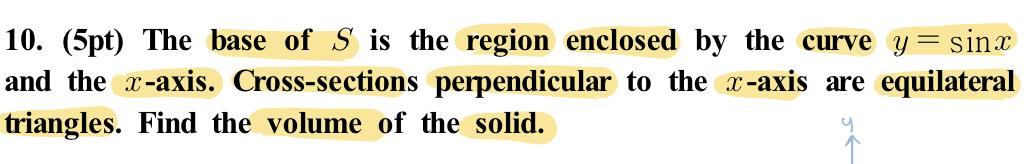### Create an Account

Already have account?

### Forgot Your Password ?

Home / Questions / 10. (5pt) The base of S is the region enclosed by the curve y=sinx and the x-axis. Cross-s...

# 10. (5pt) The base of S is the region enclosed by the curve y=sinx and the x-axis. Cross-sections perpendicular to the x-axis are equilateral triangles. Find the volume of the solid.

10. (5pt) The base of S is the region enclosed by the curve y=sinx and the x-axis. Cross-sections perpendicular to the x-axis are equilateral triangles. Find the volume of the solid.Apr 13 2021 View more View Less

#### Answer (Solved)Subscribe To Get Solution